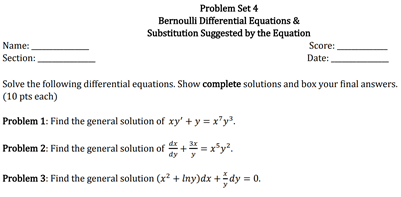### Create an Account

Already have account?

### Forgot Your Password ?

Home / Questions / Problem Set 4 Bernoulli Differential Equations & Substitution Suggested by the Equation Sc...

# Problem Set 4 Bernoulli Differential Equations & Substitution Suggested by the Equation Score: Date: Name: Section: Solve the following differential equations. Show complete solutions and box your fin

Problem Set 4 Bernoulli Differential Equations & Substitution Suggested by the Equation Score: Date: Name: Section: Solve the following differential equations. Show complete solutions and box your final answers. (10 pts each) Problem 1: Find the general solution of xy' +y = x?y? Problem 2: Find the general solution of + = x®y?. Problem 3: Find the general solution (x2 + Iny)dx +dy = 0.Apr 13 2021 View more View Less

#### Answer (Solved)Subscribe To Get Solution